# NCERT Solutions | Class 11 Physics Chapter 3 | Motion in a Straight Line## CBSE Solutions | Physics Class 11

Check the below NCERT Solutions for Class 11 Physics Part-I Chapter 3 Motion in a Straight Line Pdf free download. NCERT Solutions Class 11 Physics  were prepared based on the latest exam pattern. We have Provided Motion in a Straight Line Class 11 Physics NCERT Solutions to help students understand the concept very well.

### NCERT | Class 11 Physics Part-I

Book: National Council of Educational Research and Training (NCERT) Central Board of Secondary Education (CBSE) 11th Physics Part-I 3 Motion in a Straight Line English

#### Motion in a Straight Line | Class 11 Physics | NCERT Books Solutions

You can refer to MCQ Questions for Class 11 Physics Chapter 3 Motion in a Straight Line to revise the concepts in the syllabus effectively and improve your chances of securing high marks in your board exams.

###### Question 1.

In which of the following examples of motion, can the body be considered approximately a point object:

1. A railway carriage moving without jerks between two stations.

2. A monkey sitting on top of a man cycling smoothly on a circular track.

3. A spinning cricket ball that turns sharply on hitting the ground.

4. A tumbling beaker that has slipped off the edge of the table.

5.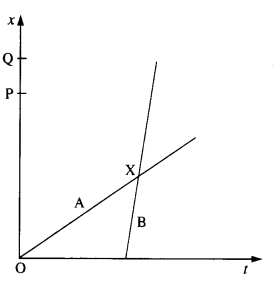1. This is an example of uniform linear motion. Hence the carriage can be considered as a point object.

2. The monkey also undergoes motion smoothly on the circular track. Hence it can be heated as a point object.

3. The ball is spinning and undergoes changes in the plane of its motion when it hits the ground. Various parts of the ball experience different forces when the ball hits the ground. It is a rigid body and cannot be treated as a point object.

4. Different parts of the beaker experience the different magnitudes of force during its motion. Hence it cannot be treated as a point object.

5. ###### Question 2.

The position-time (x-t) graphs for two children A and B returning from their school O to their homes P and Q respectively are shown in Fig.

Choose the correct entries in the brackets below:

(a) $$\frac { A }{ B }$$ lives closer to the school than $$\frac { B }{ A }$$

(b) $$\frac { A }{ B }$$ starts from the school earlier than $$\frac { B }{ A }$$

(c) $$\frac { A }{ B }$$ walks faster than $$\frac { B }{ A }$$

(d) A and B reach home at the (same/different) time.

(e) $$\frac { A }{ B }$$ overtakes $$\frac { B }{ A }$$ on the road (once/twice).

(a) A lives closer to school than B, because B has to cover higher distances [OP < OQ],

(b) A starts earlier form school than B, because t = 0 for A but for B, t has some finite time.

(c) As slope of B is greater than that of A, thus B walks faster than A.

(d) A and B reach home at the same time.

(e) At the point of intersection (i.e., X), B overtakes A on the roads once.

###### Question 3.

A woman starts from her home at 9.00 am, walks at a speed of 5 km h_1 on a straight road up to her office 2-5 km away, stays at the office up to 5.00 pm, and returns home by an auto with a speed of 25 km h_1. Choose suitable scales and plot the x-t graph of her motion.

Distance covered while walking = 2.5 km

Speed while walking = 5 km h_1

Time taken to reach office while walking =$$\frac { 2.5 }{ 5 }$$= $$\frac { 1 }{2}$$

If O is taken as the origin for both time and distance then at t = 9-00 AM, x = 0 and at t 9-30 AM, x = 2.5 km OA is the x-t graph of the motion when the woman walks from her home to office. She stays in the office from 9-30 AM to 5-00 PM and is represented by the straight line AB.

Now time taken to return home = $$\frac { 2.5 }{ 5 }$$= $$\frac { 1 }{ 10 }$$

h = 6 minutes

So at 5. 06 PM, x = 0

This is represented by the line BC in the graph.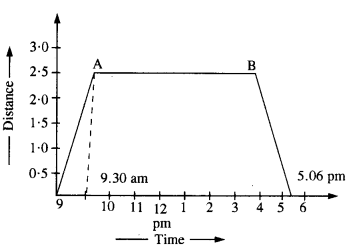###### Question 4.

A drunkard walking in a narrow lane takes 5 steps forward and 3 steps backward, followed again by 5 steps forward and 3 steps backward, and so on. Each step is 1m long and requires 1 s. Plot the x-t graph of his motion. Determine graphically and otherwise how long the drunkard takes to fall in a pit 13 m away from the start.

Distance travelled in 5s = 5 m

Distance travelled in 8s=5-3=2m

Distance travelled in 13 s = 2 + 5 = 7m

Distance travelled in l6s = 7- 3 =4m

Distance travelled in 21s = 4 + 5 = 9m

Distance travelled in 24s = 9- 3 =6m

Distance travelled in 29s = 6 + 5 = 1m

Distance travelled in 32s=11-3 = 8m

Distance travelled in 37s=8 + 5 =13m

Since each step requires one second of time therefore total time is 37 seconds. The graph is as shown.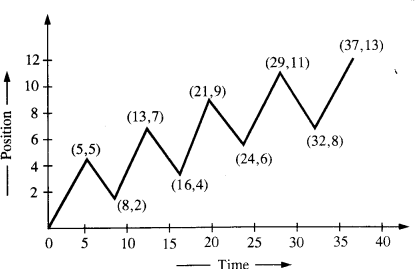###### Question 5.

A jet airplane travelling at the speed of 500 km h_1 ejects its products of combustion at the speed of 1500 km h_1 relative to the jet plane. What is the speed of the latter with respect to an observer on the ground?

Speed of the exhaust with respect to an observer on the ground = Speed of exhaust with respect to the plane – Speed of plane with respect to the ground, (minus sign because the plane and exhaust move in opposite directions)

= (1500 – 500) km h-1

= 1000 km h-1

###### Question 6.

A car moving along a straight highway with a speed of 126 km h_1 is brought to a stop within a distance of 200 m. What is the retardation of the car (assumed uniform) and how long does it take for the car to stop?

u = 126 km h 1 = 126 x$$\frac { 5 }{ 18 }$$=35 ms_1

S = 200 m and υ = 0

υ2– u2 = 2 a S

∴ 0 – (35)2 = 2a x 200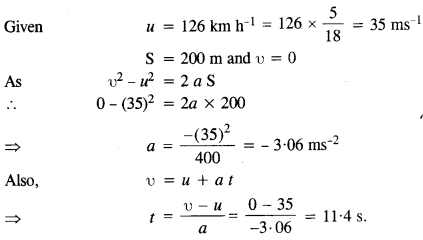###### Question 7.

Two trains A and B of length 400 m each are moving on two parallel tracks with a uniform speed of 72 km h-1 in the same direction, with A ahead of B. The driver of B decides to overtake A and accelerates by 1 m s-2. If after 50 s, the guard of B just brushes past the driver of A, what was the original distance between them?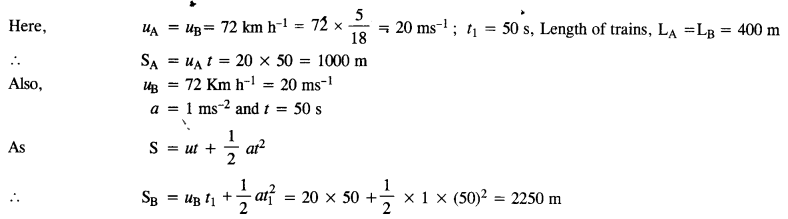∴ Original distance between trains = (Sb – SA)-((LA + LB) = (2250 – 1000) – (800) = 450 m.

###### Question 8.

On a two-lane road, car A is travelling at a speed of 36 km h_1. Two cars B and C approach car A in opposite directions with a speed of 54 km h_1 each. At a certain instant, when the distance AB is equal to AC, both being 1 km, B decides to overtake A before C does. What minimum acceleration of car B is required to avoid an accident?###### Question 9.

Two towns A and B are connected by regular bus service with a bus leaving in either direction every T minutes. A man cycling with a speed of 20 km h_1 in the direction A to B notices that a bus goes past him every 18 min, in the direction of his motion, and every 6 min in the opposite direction. What is the period T of the bus service and with what speed (assumed constant) do the buses ply on the road?

Let υb = speed of each bus and υc = speed of cyclist

Relative speed of buses plying in the same direction of motion of cyclist = υb – υc

The buses plying in the direction of motion of the cyclist go past him after every 18 minutes i.e., $$\frac { 18 }{ 60 }$$ h

.’. Distance covered by each bus is (υb – υc) x $$\frac { 18 }{ 60 }$$

Since a bus leaves after every T minute therefore distance is also equal to υb x $$\frac { T }{ 60 }$$

.’. (υb – υc) x $$\frac { 18 }{ 60 }$$ = υb x $$\frac { T }{ 60 }$$

Relative velocity of the buses plying opposite to the direction of motion of the cyclist is υb + υc after every 6 minutes.

.’. Distance covered by each bus is (υb + υc) x $$\frac { 6 }{ 60 }$$###### Question 10.

A player throws a ball upwards with an initial speed of 294 ms_1.

(a) What is the direction of acceleration during the upward motion of the ball?

(b) What are the velocity and acceleration of the ball at the highest point of its motion?

(c) Choose the x = 0 m and t = 0 s to be the location and time of the ball at its highest point, vertically

downward direction to be the positive direction of the x-axis, and give the signs of position, velocity, and acceleration of the ball during its upward and downward motion.

(d) To what height does the ball rise and after how long does the ball return to the player’s hands? (Take g=98 m s_2 and neglect air resistance).

(a) The ball is under the influence of acceleration due to gravity which always acts vertically downwards.

(b) Velocity at the highest point = zero

Acceleration at highest point = g = 98 ms_2 (vertically downwards)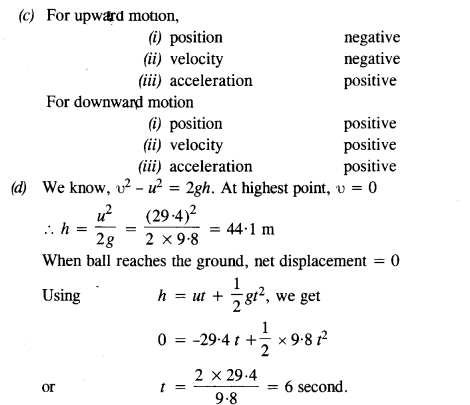###### Question 11.

Read each statement below carefully and state with reasons and examples, if it is true or false:

A particle in one-dimensional motion

1. with zero speed at an instant may have non-zero acceleration at that instant

2. with zero speed may have non-zero velocity. ‘

3. with constant speed must have zero acceleration.

4. with a positive value of acceleration must be speeding up.

1. True. Consider a ball thrown vertically, upward. At the highest point, the speed is zero but the acceleration of the ball is non-zero ( 9.8ms-2 vertically downwards). Acceleration does not depend on instantaneous speed.

2. False. Since the magnitude of velocity is speed, a body with zero speed must have zero velocity.

3. True. In the case of a body rebounding with the same speed, the acceleration at the time of impact is infinite, which is not practical physically.

4. False. This depends on the chosen positive direction. The statement is true when the direction of motion and acceleration is along the chosen positive direction.

5. ###### Question 12.

A ball is dropped from a height of 90 m on a floor. At each collision with the floor, the ball loses one-tenth of its speed. Plot the speed-time graph of its motion between t = 0 to 12 s.###### Question 13.

Explain clearly, with examples, the distinction between:

(a) the magnitude of displacement (sometimes called distance) over an interval of time, and the total length of path covered by a particle over the same interval;

(b) the magnitude of average velocity over an interval of time, and the average speed over the same interval [Average speed of a particle over an interval of time is defined as the total path length divided by the time interval]. Show in both (a) and (b) that the second quantity is either greater than or equal to the first. When is the equality sign true ? [For simplicity, consider one-dimensional motion only].

(a) Magnitude of displacement over an interval of time may be zero, whereas the total length of the path covered by the particle over the same interval is not zero. For example, consider a particle moving along a straight line from point A to point B distant S from each other and then back to point A in time interval t as shown in fig.In this case, magnitude of displacement of the particle over an interval of time t = 0.

Total length of the path covered by the particle over the interval of time t = AB + BA = 2 S

(b)(c) In both the cases (a) and (b), the second quantity is greater than the first quantity e. The total length of path > magnitude of displacement and average speed > magnitude of average velocity. If the direction of motion of a particle along a straight line does not change, the the magnitude of displacement of the particle over a time interval = Total length of the path covered by the particle over the same time interval and magnitude of velocity = speed of the particle.

###### Question 14.

A man walks on a straight road from his home to a market 2.5 km away with a speed of 5 km h-1. Finding the market closed, he instantly turns and walks back home with a speed of 7.5 km h-1. What is the

(a) the magnitude of average velocity, and

(b) the average speed of the man over the interval of time

(1) 0 to 30 min,

(2) 0 to 50 min,

(3) 0 to 40 min?

[Note: You will appreciate from this exercise why it is better to define average speed as total path length divided by time, and hot as the magnitude of average velocity. You would not like to tell the tired man on his return home that his average speed was zero !]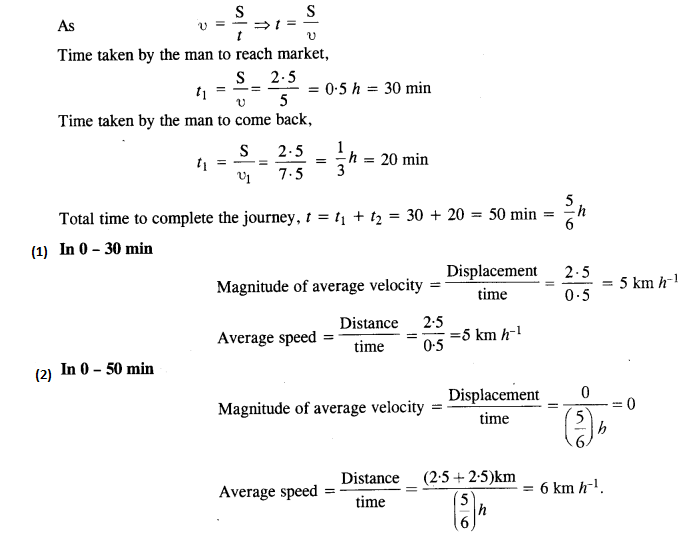(3) In 0-40 min

In 0 – 30 min. man goes from home to market with a sped of 5 km h’. In the next 10 mm, the man goes from the market towards home with speed of 75 km h-1 Distance travelled by man in these 10 min = speed x time = 75 km h-1x $$\frac { 10 }{ 60 }$$ h###### Question 15.

In 13 and 14, we have carefully distinguished between average speed and magnitude of average velocity. No such distinction is necessary when we consider the instantaneous speed and the magnitude of velocity. The instantaneous speed is always equal to the magnitude of instantaneous velocity. Why?

The instantaneous speed is always equal to the magnitude of the instantaneous velocity because for very small instants of time the length of the path is equal to the magnitude of displacement.

###### Question 16.

Look at the graphs (a) to (d) (Fig.) carefully and state, with reasons, which of these cannot possibly represent one- dimensional motion of a particle.(a) From the graph, we see that for certain instants of time, the particle has 2 positions, which is not possible. Hence this graph cannot possibly represent the one-dimensional motion of a particle.

(b) From the graph, we see that for certain instants of time, the particle has 2 velocities, which is not possible. Hence this graph cannot possibly represent the one-dimensional motion of a particle.

(c) From the graph, we see that for some instants of time, the particle has negative speed. But speed is always a non-negative quantity. Hence this graph cannot possibly represent the one-dimensional motion of a particle.

(d) From the graph, we see that for a time interval the total path length is decreasing. But total path length is always a nondecreasing quantity. Hence this graph cannot possibly represent the one-dimensional motion of a particle.

###### Question 17.

Figures show the x-t plot of the one-dimensional motion of a particle. Is it correct to say from the graph that the particle moves in a straight line for t < 0 and on a parabolic path for t > 0? If not, suggest a suitable physical context for this graph.For t < 0, x = 0, so particle is at rest and not moving in a straight line,

For t > 0, a particle can move on a parabolic path if its acceleration is constant.Therefore, it is not correct to say from graph that the particle moves in a straight line for r < 0 and on a parabolic path for t > 0.

For the graph, a suitable physical context can be the particle thrown from the top of a tower at the instant t = 0.

###### Question 18.

A police van moving on a highway with a speed of 30 km he fires a bullet at their car speeding away in the same direction with a speed of 192 km h-1. If the muzzle speed of the bullet is 150 ms-1, with what speed does the bullet hit their car? (Note: Obtain that speed which is relevant for damaging their car).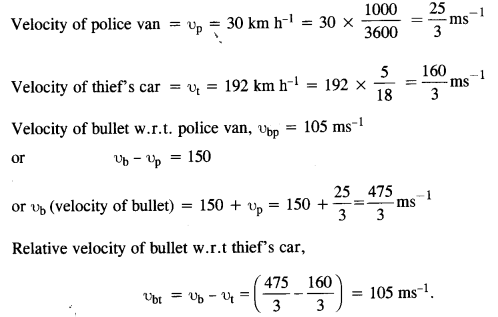###### Question 19.

Suggest a suitable physical situation for each of the following graphs.(a) consider a ball which is pushed at some time t < 0 towards a wall. Upon rebounding from this wall it hits the opposite wall and comes to a stop. If x = 0 for the initial position, then this context may have the given x -t graph.

(b) Consider a ball thrown vertically upwards, with the vertically upward direction chosen as the positive direction. Each time it hits the ground, it loses a fraction of its velocity and finally comes to rest. The ball may be represented in the given v -t graph in this context.

(c) Consider a cricket ball moving with a uniform velocity which is hit by the bat and then turns back. In this case, the a-t graph for the ball may be similar to the one given above.

###### Question 20.

The figure gives the x-t plot of a particle executing one-dimensional simple harmonic motion. (You will learn about this motion in more detail in Chapter 14). Give the signs of position, velocity, and acceleration variables of the particle at t = 0.3 s, 1.2 s, -1.2 s.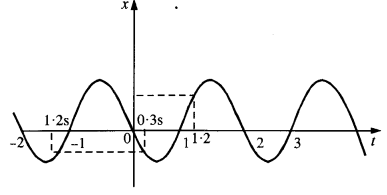(1) At t = 0-3 s, x is -ve. Velocity = slope of x -t graph.

Since slope of x -t is negative, so velocity is negative. In simple harmonic motion, the direction of acceleration is opposite to the direction of displacement of the particle, so acceleration is positive.

(2) At t = 1.2 s, x = + ve. v is also +ve as slope of x -t graph is + ve. Acceleration a is -ve

(3) At t = -1.2 s, x =-ve. so a is +ve, v =Δx/Δt = +ve as both Δx and Δt are negative.

###### Question 21.

The figure gives the x-t plot of a particle in one-dimensional motion. Three different equal intervals of time are shown. In which interval is the average speed greatest, and in which is it the least ? Give the sign of average velocity for each interval.

The magnitude of the slope of the x – t graph is highest in 3 and least in 2. Hence, the average speed is greatest in 3 and least in 2. Also, the sign of the slope of the x -t graph is positive for 1 and 2 and negative for 3. Therefore sign of the average velocity ‘V’ is:-

v > 0 for intervals 1 and 2

v < 0 for intervals 3.###### Question 22.

The figure gives a speed-time graph of a particle in motion along a constant direction. Three equal intervals of time are shown. In which interval is the average acceleration greatest in magnitude? In which interval is the average speed greatest? Choosing the positive direction as the constant direction of motion, give the signs of o and a in the three intervals. What are the accelerations at points A, B, C and D?Acceleration magnitude is greatest in 2 because slope of υ -t graph at this interval is maximum.

Average speed is greatest in 3.

υ>0 in 1, 2 and 3\a > 0 in 1, a < 0 in 2, a = 0 in 3.

Acceleration is zero at A, B, C and D because slope of υ-t graph at these points is zero.

###### Question 23.

A three-wheeler starts from rest, accelerates uniformly with 1 m s-2 on a straight road for 10 s, and then moves with uniform velocity. Plot the distance covered by the vehicle during the nth second (n = 1, 2, 3….) versus n. What do you expect this plot to be during accelerated motion: a straight line or a parabola?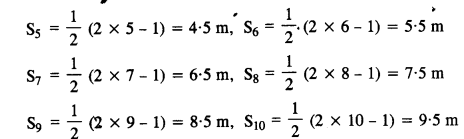###### Question 24.

A boy standing on a stationary lift (open from above) throws a ball upwards with the maximum initial speed he can, equal to 49 m s_1. How much time does the ball take to return to his hands ? If the lift starts moving up with a uniform speed of 5 m s_1 and the boy again throws the ball up with the maximum speed he can, how long does the ball take to return to his hands ?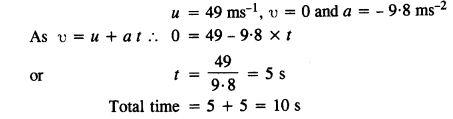When lift is moving upward with uniform velocity, the initial velocity of the ball will remain 49 ms-1 only w.r.t lift. Thus the time take up by ball will be 10 s.

###### Question 25.

On a long horizontally moving belt (Fig.), a child runs to and fro with a speed of 9 km h_1 (with respect to the belt) between ms father and mother located 50 m apart on the moving belt. The belt moves with a speed of 4 km h_1. For an observer on a stationary platform outside, what is the

(a) speed of the child running in the direction of motion of the belt?

(b) speed of the child running opposite to the direction of motion of the belt?

(c) time is taken by the child in (a) and (b)?

Which of the answers alter if motion is viewed by one of the parents?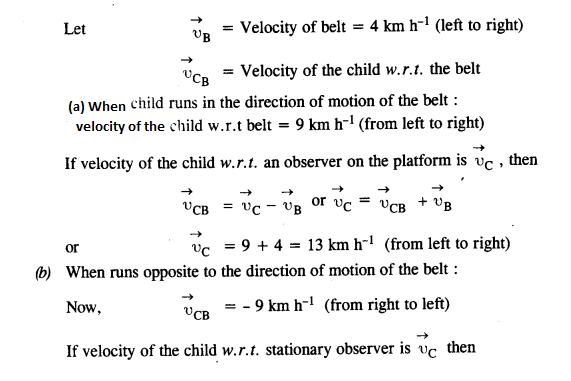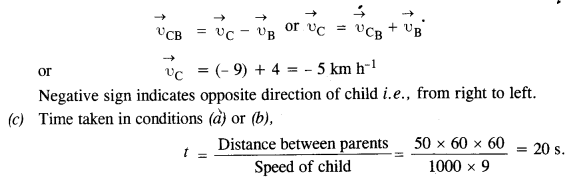###### Question 26.

Two stones are thrown up simultaneously from the edge of a cliff 200 m high with initial speeds of 15 ms-1 and 30 ms-1. Verify that the graph shown in Fig. correctly represents the time variation of the relative position of the second stone with respect to the first. Neglect air resistance and assume that the stones do not rebound after hitting the ground. Take g = 10 ms-2. Give the equations for the linear and curved parts of the plot.For maximum separation, t = 8 s

So maximum separation is 120 m

After 8 seconds, only the second stone would be in motion. Its motion is described by eqn.(ii) So, the graph is in accordance with the quadratic equation.

###### Question 27.

The speed-time graph of a particle moving along a fixed direction is shown in

Fig. Obtain the distance traversed by the particle between

(a) t = 0 s to 10 s.

(b) t = 2 s to 6 s.

What is the average speed of the particle over the intervals in (a) and (b)?(a) Distance travelled from t = 0 to t = 10 s

= area under speed time graph,

= $$\frac { 1 }{ 2 }$$ x 10 x 12 = 60m

(b) Average speed over the interval from t = 0 to t = 10 s is $$\frac { 60 }{ 10 }$$ =6 ms-1

(c) In order to calculate distance from t = 2 s to t = 6 s, let us first determine separately the distance covered from t

= 2 s to r = 5 s and the distance covered form t = 5 s to t = 6 s, then add.

12

(i) Acceleration =$$\frac { 12 }{ 5 }$$ = 24 ms2

Velocity at the end of 2 s = 24 X 2 = 4.8 ms-1.###### Question 28.

The velocity-time graph of a particle in one-dimensional motion is shown in Fig.

Which of the following formulae are correct for describing the motion of the particle over the time-interval t1 to t2 :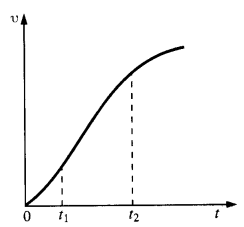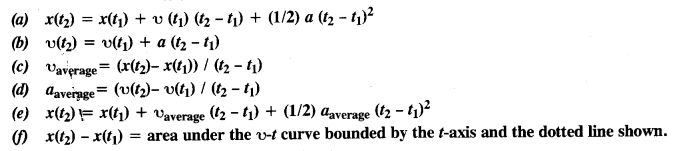(a) This formula is not correct as it is applicable only if a is constant. In time interval t1 to t2, a is not constant.

(b) This formula is not correct as it is applicable only if a is In time-interval t1 to t2, a is not constant.

(c) and (d) are correct. They represent the definitions of υav and υav.

(d) This formula is not correct as such formula does not contain uav and aav.

(e) This formula is correct because of the area under υ-t graph = displacement of a particle.